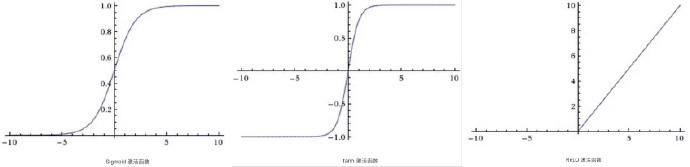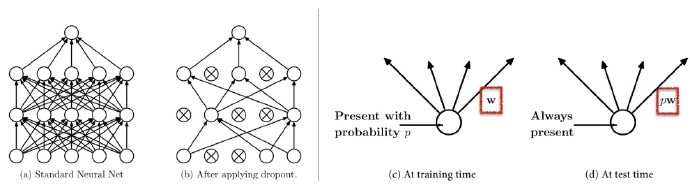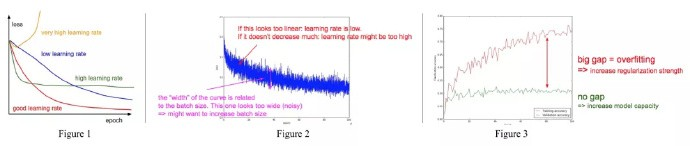• 数据增广
• 图像预处理
• 网络初始化
• 训练过程中的技巧
• 激活函数的选择
• 不同正则化方法
• 来自于数据的洞察
• 集成多个深度网络的方法.

(部分有点小乱.)

## 2. 预处理

### 2.1 最简单的预处理方法

• 零均值化
• 标准化

#### 2.1.2 为什么要归一化

$$S = w_1 * x_1 + w_2 * x_2 +b$$

$$\frac{d S}{d w_1} = x_1; \frac{d S}{d w_2} = x_2$$

#### 2.1.3. 实现代码

X-=numpy.mean(X,axis=0) # 即X的每一列都减去该列的均值

X-=numpy.mean(X)
X/=numpy.std(X,axis=0) # 数据归一化. 

X是输入数据，(图片数目X图片维度).

### 2.2 白化(Whitening)

X-=np.mean(X,axis=0)
cov=np.dot(X.T,X)/X.shape #计算协方差矩阵

U,S,V=np.linalg.svd(cov)
#计算数据协方差矩阵的奇异值分解(SVDfactorization)
Xrot=np.dot(X,U)
#对数据去相关

Xwhite=Xrot/np.sqrt(S+1e-5) #除以奇异值的平方根，
# 注意到这里加了个1e-5是为了防止分母是0的情况

PCA 白化的一个缺点是会增加数据中的噪声，因为它把输入数据的所有维度都延伸到相同的大小，这些维度中就包含噪音维度(往往表现为不相关的且方差较小). 这种缺点在实际操作中可以通过把1e-5增大到一个更大的值来引入更强的平滑.

## 3. 初始化

### 3.2. 用小的随机数初始化

W = 0.01 * numpy.Random.randn(D,H)

randn方法生成一个零均值，方差为1的正态分布的随机数，同样也可以换用数值较小的均匀分布来产生初始化参数，但在实践中两种方法最终结果差别不大.

### 3.3. 方差归一化

$$Var(A + B + C) = Var(A) + Var(B) + Var(C)$$

$$S = w_1 * x_1 + w_2 * x_2 +...+ w_i * x_i + b$$

S 是多个随机变量的加权和, 假设W各维度之间相互独立，随着数据维度增长，S的方差将会线性积累，由于数据的维度随任务的不同，是不可控的，所以我们希望将S的方差做归一化，这只需要对 W 做处理就可以了：

W=numpy.random.randn(n)/sqrt(n)  #n是数据维度

w = numpy.random.randn(n) * sqrt(2.0/n)

## 5. 激活函数

sigmoid 与 tanh 曾经很流行，但现在很少用于视觉模型了，主要原因在于当输入的绝对值较大时，其梯度(导数)接近于零，这时参数几乎不再更新，梯度的反向传播过程将被中断，出现梯度消散的现象.ReLU 优点：

• 实现起来非常简单，加速了计算过程.
• 加速收敛，没有饱和问题，大大缓解了梯度消散的现象.

ReLU 缺点：

$$F ＝ w1*x1 + w2*x2$$

• Leaky ReLU 就是直接给 alpha 指定一个固定的值，整个模型都用这个斜率：
• Parametric ReLU 将 alpha 作为一个参数，通过从数据中学习获取它的最优值.
• Randomized ReLU 的 alpha 是在规定的区间内随机选取的，在测试阶段是定值.

## 6. 正则化

### 6.1. L2正则化

L2正则化也许是最常用的正则化的形式.

### 6.2. L1正则化

L1正则化是另一个相关的常见的正则化方式.

L1正则化有一个非常有趣的属性，那就是它会使得权重向量 w 在优化期间变得稀疏(例如非常接近零向量).

### 6.4. Dropout

Dropout 是一个极其有效的、简单的并且是最近才被提出的正则化技术作为以上三种正则化方法(L1、L2、最大范数约束)的补充.## 7. 从数字中观察Figure 1 中，

### 7.2. 放大loss曲线观察

Figure 2 中横坐标是epoch(网络在整个训练集上完整的跑一遍的时间，所以每个epoch中会有多个mini batches)，纵坐标是每个训练batch的分类loss.

### 7.3. 从精确率曲线观察

Figure 3 中红色线是训练集上的精确率，绿色验证集上的精确率.

## 8. 集成

### 8.3. 单个模型的不同检查点

Last modification：January 18th, 2019 at 02:10 pm